# Printable Math Addition Drill 13OtherMar 14, 2021By PrintableeNo Comment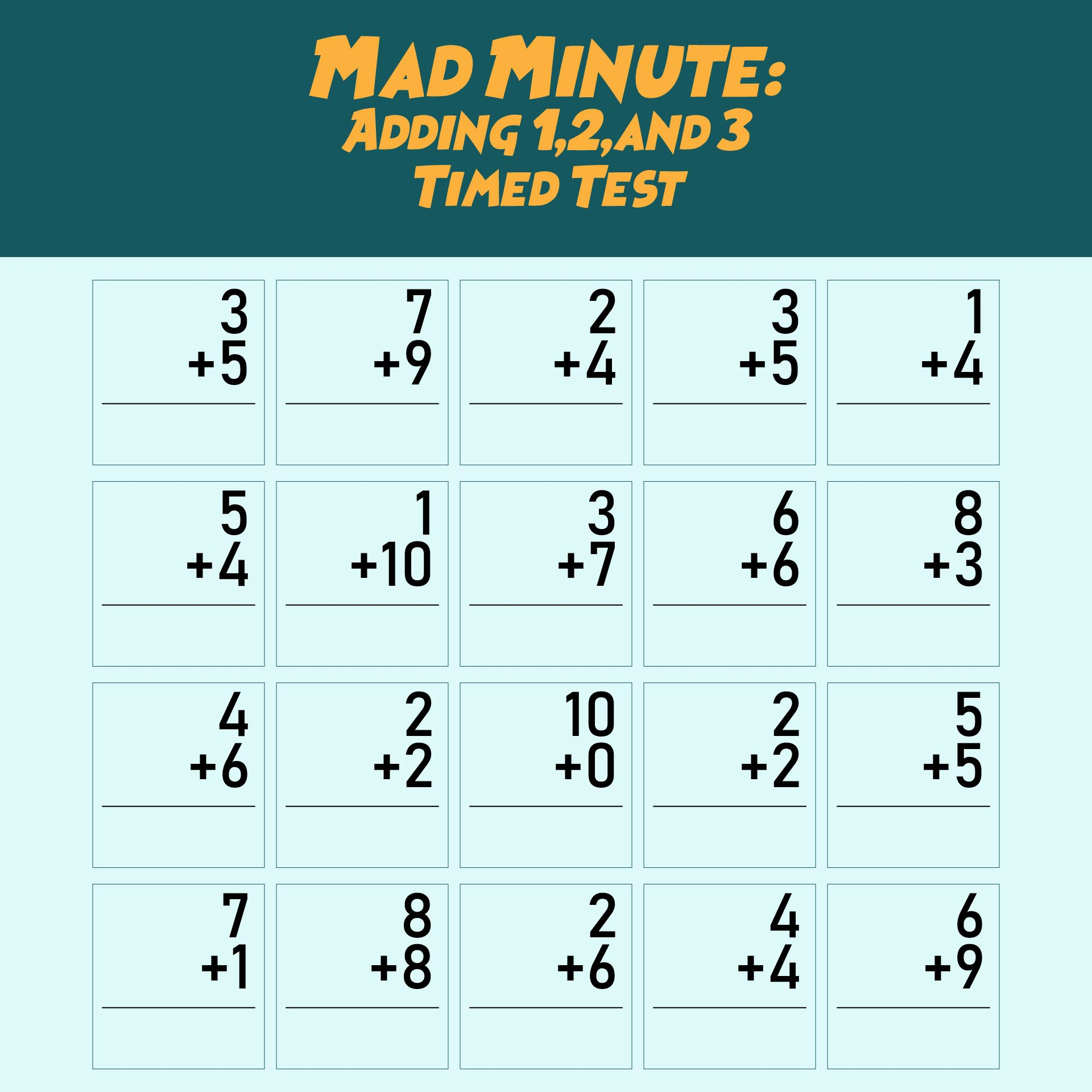### What Kind of Math is Addition?

The addition is one of the basic math operations usually represented by the symbol '+'. In basic math, there are many ways of saying the same thing. For example, the symbol '+' can be called addition, add, sum, plus, increase, or total. The addition is a math operation that brings two or more numbers (or things) together to make a new total. The numbers added together are called 'addends'. An addition operation can be as simple as two plus two equals four, but also can be more complicated if applied to more complicated math operations.

### What are the Addition Strategies?

There are lots of thinking tricks that make addition easier. For instance, it is easier to start from the larger number. For example, it is easier to count 6+3 than 3+6 for there are fewer numbers to count upwards. You can also use "jump strategy". By using this strategy, you don't count up one by one but by 2s or 10s. It will make the counting process a lot faster. You can also add up to ten by seeing if any numbers add to ten. They don't have to be next to each other. Add the first, and add the rest of the numbers. You can also split the bigger number into tens and units to make the addition easier.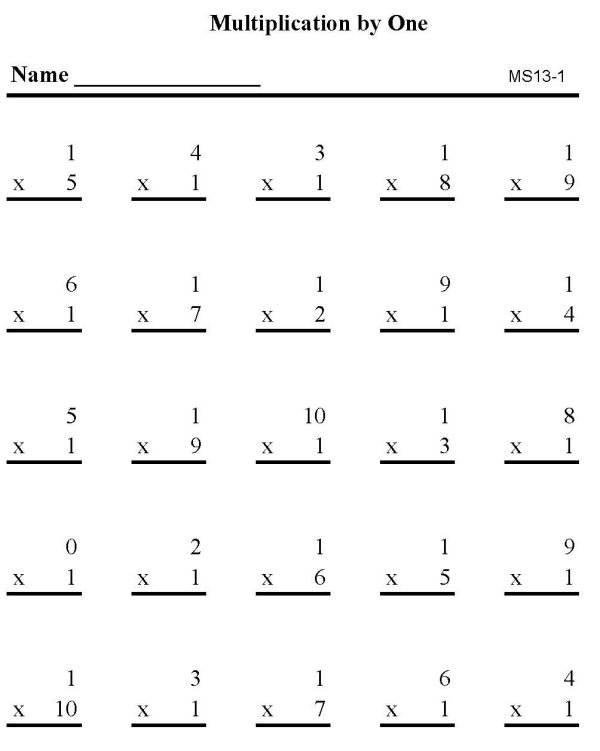We also have more printable other you may like:
Printable Timed Math Drills Multiplication
Printable New Year's Eve
Printable Diet For Ibs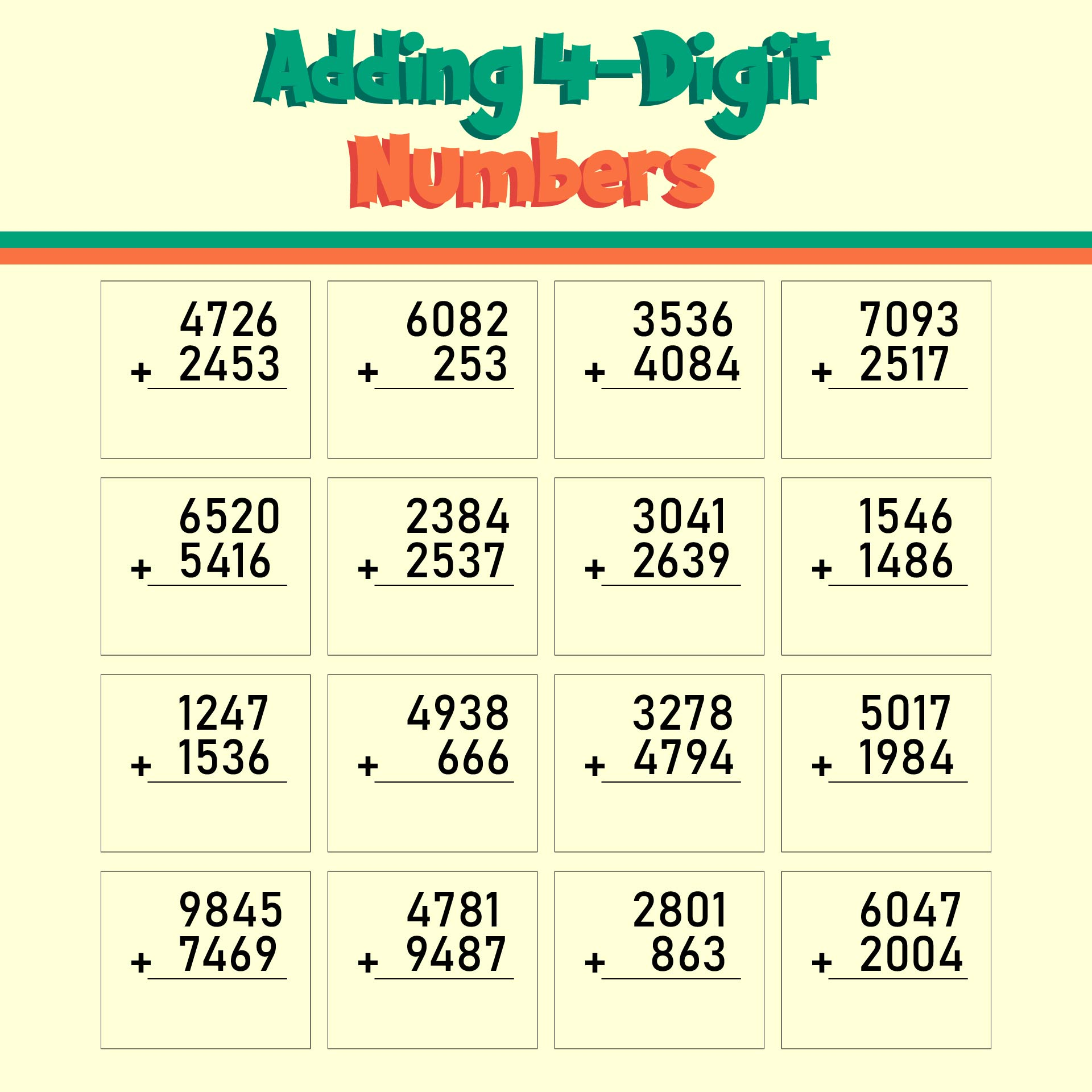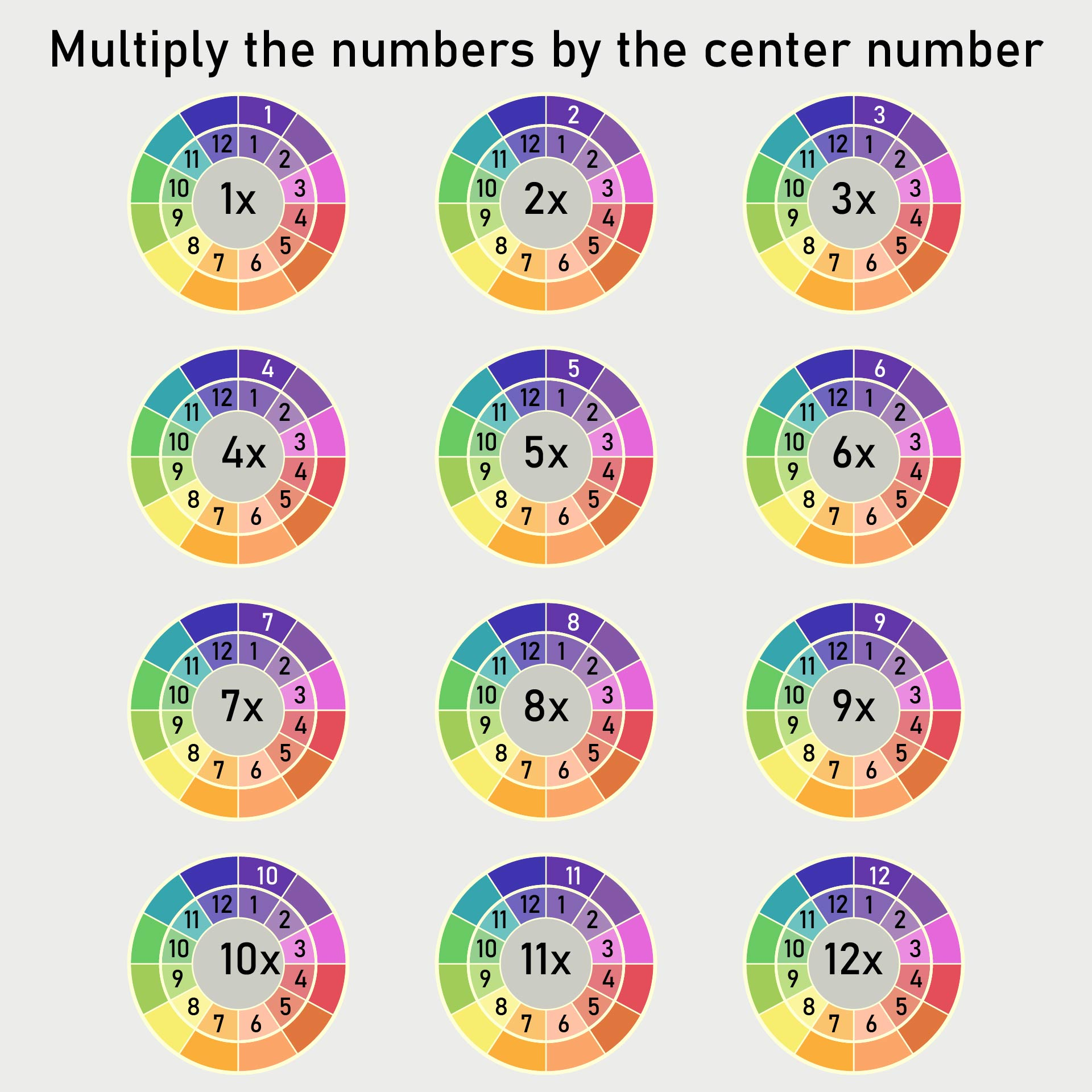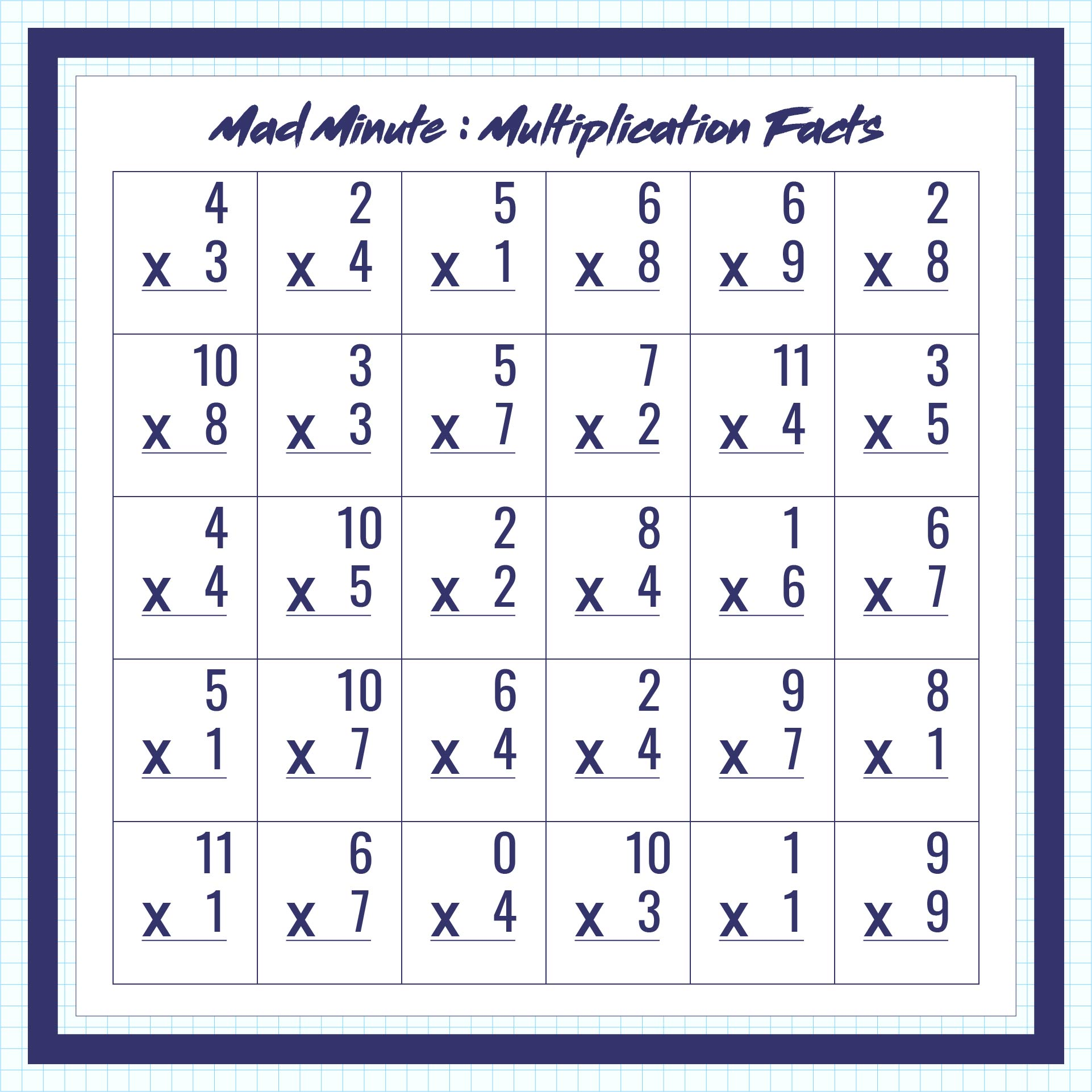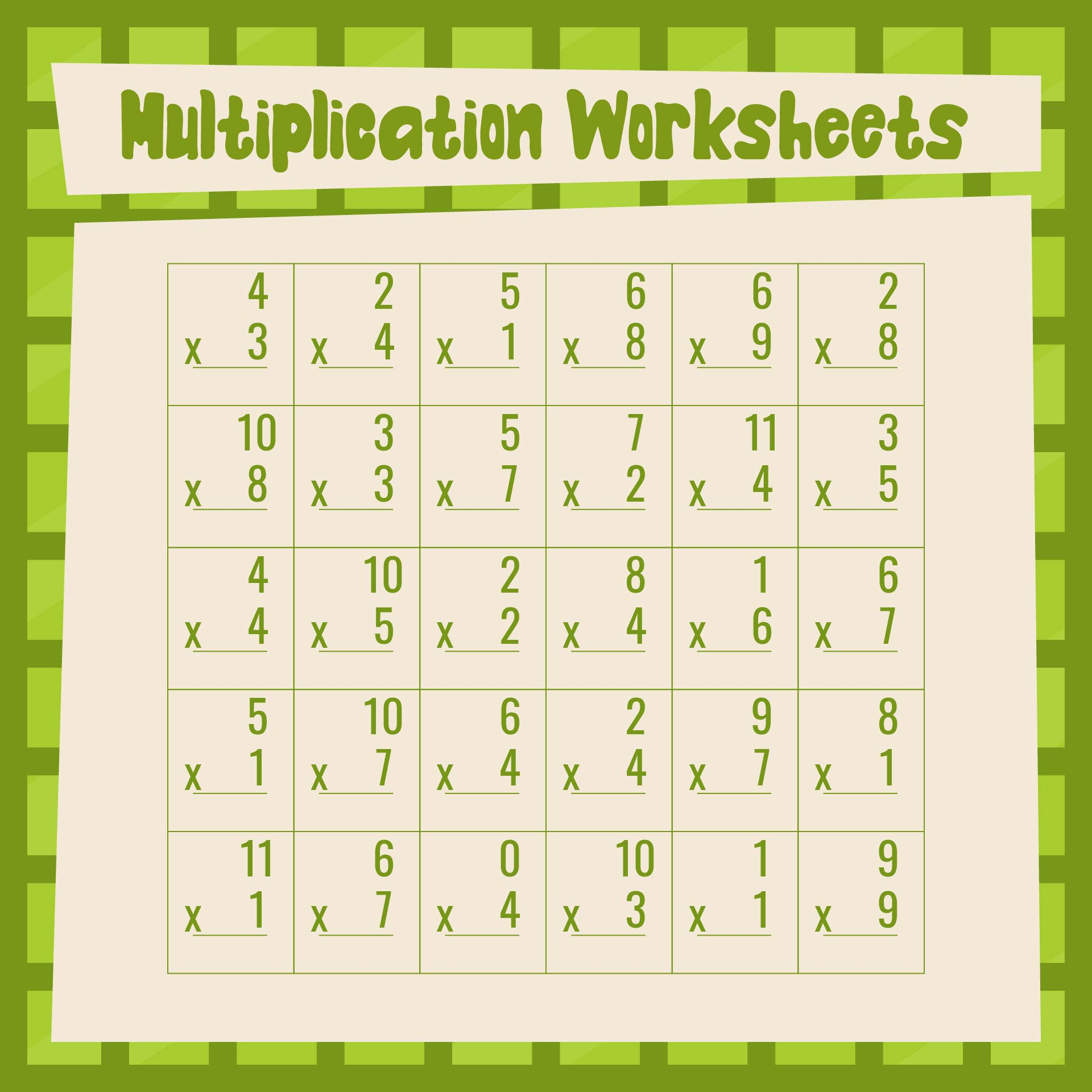### What is the Easiest Way to Learn Addition?

There are a lot of easy ways to learn addition. One of them is using games. The learning process can be a lot easier and fun when combined with games. Here are some of the games you can try to learn addition:
1.    The Dice Game
For this game, you will need a pair of dice. Then ask your friends, siblings, or parents to give you a number. For example, if they give you number 6, you can try to pair the dice that equals 6: 5+1, 4+2, 3+3, and so on. You can see various possibilities of addition here. You can use more dice to learn a bigger number.
2.    Using Pebbles and Blackboard
You can create a fun addition using pebbles and blackboard. First, you must think of a number, for example, three. Place three pebbles on the table and write the number three on the board. And then, think of another number, for example, two. Add two pebbles on the table and write it on the board including a '+' symbol between them. Now count the pebbles on the table and write the result on the board.